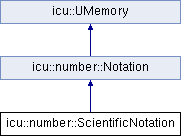ICU 71.1  71.1
icu::number::ScientificNotation Class Reference

A class that defines the scientific notation style to be used when formatting numbers in NumberFormatter. More...

`#include <numberformatter.h>`

Inheritance diagram for icu::number::ScientificNotation:## Public Member Functions

ScientificNotation withMinExponentDigits (int32_t minExponentDigits) const
Sets the minimum number of digits to show in the exponent of scientific notation, padding with zeros if necessary. More...

ScientificNotation withExponentSignDisplay (UNumberSignDisplay exponentSignDisplay) const
Sets whether to show the sign on positive and negative exponents in scientific notation. More...

## Friends

class Notation

class impl::NumberPropertyMapperStatic Public Member Functions inherited from icu::number::Notation
static ScientificNotation scientific ()
Print the number using scientific notation (also known as scientific form, standard index form, or standard form in the UK). More...

static ScientificNotation engineering ()
Print the number using engineering notation, a variant of scientific notation in which the exponent must be divisible by 3. More...

static CompactNotation compactShort ()
Print the number using short-form compact notation. More...

static CompactNotation compactLong ()
Print the number using long-form compact notation. More...

static SimpleNotation simple ()
Print the number using simple notation without any scaling by powers of ten. More...

## Detailed Description

A class that defines the scientific notation style to be used when formatting numbers in NumberFormatter.

To create a ScientificNotation, use one of the factory methods in Notation.

Stable:
ICU 60

Definition at line 408 of file numberformatter.h.

## ◆ withExponentSignDisplay()

 ScientificNotation icu::number::ScientificNotation::withExponentSignDisplay ( UNumberSignDisplay exponentSignDisplay ) const

Sets whether to show the sign on positive and negative exponents in scientific notation.

The default is AUTO, showing the minus sign but not the plus sign.

For example, with exponentSignDisplay=ALWAYS, the number 123 will be printed as "1.23E+2" in en-US instead of the default "1.23E2".

Parameters
Returns
A ScientificNotation, for chaining.
Stable:
ICU 60

## ◆ withMinExponentDigits()

 ScientificNotation icu::number::ScientificNotation::withMinExponentDigits ( int32_t minExponentDigits ) const

Sets the minimum number of digits to show in the exponent of scientific notation, padding with zeros if necessary.

Useful for fixed-width display.

For example, with minExponentDigits=2, the number 123 will be printed as "1.23E02" in en-US instead of the default "1.23E2".

Parameters
 minExponentDigits The minimum number of digits to show in the exponent.
Returns
A ScientificNotation, for chaining.
Stable:
ICU 60

The documentation for this class was generated from the following file: